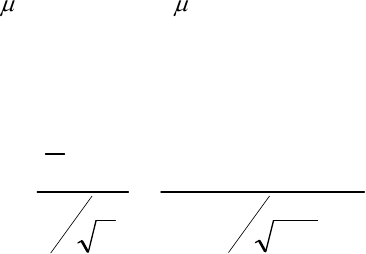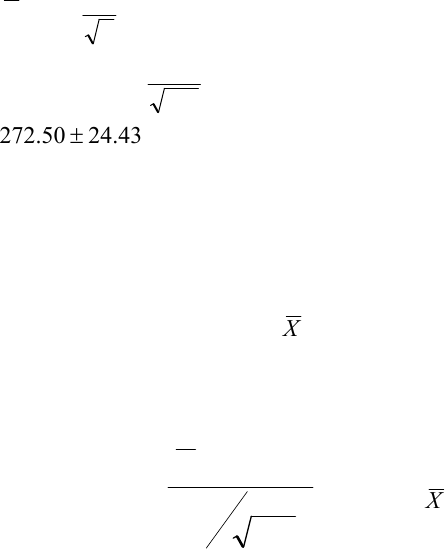Class Notes (1,100,000)
CA (650,000)
UTSC (30,000)
ATA (10)
Lecture 1

# MGEB12H3 Lecture Notes - Lecture 1: Null Hypothesis, Confidence Interval, Test StatisticExam

Department
Economics for Management Studies
Course Code
MGEB12H3
Professor
ATA
Lecture
1

This preview shows page 1. to view the full 5 pages of the document.Page 1 of 5
TUTORIAL-1
Long Question: The manager of a department store is thinking about establishing a new
billing system for the store's customers. After a thorough financial analysis, she
determines that the new system will be cost-effective only if the mean monthly account
balance is greater than \$250. A random sample of 111 monthly accounts is drawn. The
sample mean is \$272.50, and the sample standard deviation is \$130.00.
a) Identify the Null and Alternative hypotheses for a hypothesis test that would allow
the manager to decide whether there is sufficient evidence to conclude that the new
system will be cost-effective. Using a value of α = .05, identify the critical value(s) of
the test statistic used for this hypothesis test. State your conclusion.
Solution: H0:
= 250 H1:
> 250
Test Statistic: t =
8235.1
111
130
00.25050.272
0
n
S
X
Decision: Reject the null hypothesis.
Conclusion: There is enough evidence to indicate that the new system is cost effective.
b) Using the t-table show how you can approximate the p-value of your test. in (a).
Solution:
0.025<p-value <0.05
the t-stat of 1.8235 is between 1.658, 1.980 from the table.
c) Calculate the 95% confidence interval for the population mean. Briefly explain the
meaning of the confidence interval. Based on this confidence interval redo your test
in (a).
Solution:

Unlock to view full version

Only page 1 are available for preview. Some parts have been intentionally blurred.Page 2 of 5
43
.
24
50
.
272
111
130
98.150.272
2/
n
s
tX
The null as appeared in (a) is inside the confidence interval implying that the
null cannot be rejected at 2.5%, (Note: this is a one sided test)). We cannot
reject at 2.5% but that does not imply we cannot reject at 5%. Therefore based
on the confidence interval alone we cannot do the test in (a).
d) Find the smallest value for
X
that allows you to reject the null in (a).
Answer: To reject the null hypothesis, we would need to see a test statistic that is more
extreme than the critical value of 1.6588:
Test Statistic: t =
111
130
00.250X
= 1.6588;
X
= 270.47